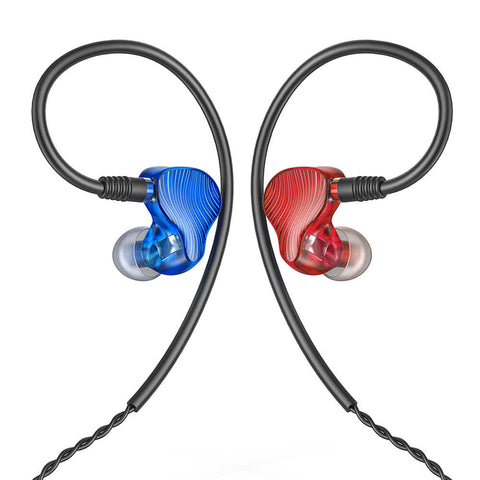# FiiO FA1 Single Balanced Armature In-Ear Monitors

• Rs. 8,990.00
• Save Rs. 1,000

## .sold_pro{ color: #f18d00; } label.sold.sold_pro,.pro-inventory{ display: block;margin-bottom:5px; } .emoticon{vertical-align: top;} .hide{display:none;} .inline{display:inline;} b{font-weight:bold;} label.sold.sold_pro,.pro-inventory{display:inline;} function getParameterByName(name) { name = name.replace(/[\[]/, "\\[").replace(/[\]]/, "\\]"); var regex = new RegExp("[\\?&]" + name + "=([^&#]*)"), results = regex.exec(location.search); return results === null ? "" : decodeURIComponent(results.replace(/\+/g, " ")); } var last_id = '0'; window.onload = function() { var limit = 50; if(\$(".sold_box_sub").hasClass("for_product")){ /* Total Product In stock */ var total_product=0; \$(".pro_stock").each(function(){ total_product = parseInt(total_product) + parseInt(\$(this).val()); }); if(limit < total_product){ total_product = 0; } if(total_product >0){ \$(".pro-inventory").prepend('<b>'+total_product+'</b>'); \$(".pro-inventory").show(); \$('.separator').show(); } /* end */ } if(\$(".sold_box_sub").hasClass("for_variant")){ var variantId_in_URL = getParameterByName("variant"); variantId_in_URL = \$.trim(variantId_in_URL); if(variantId_in_URL == ""){ var variantId = \$('#selectedVariant').val(); } else { variantId = variantId_in_URL; } /* check first variant is available or not */ if(\$(".sa_"+variantId).length == 1){ var inv = \$(".sa_"+variantId).attr('inv'); if(limit < inv) \$(".sa_"+variantId).addClass('hide'); } if(\$(".sa_"+variantId).length == 1 && !\$(".sa_"+variantId).hasClass('hide')){ \$(".sa_"+variantId).show(); last_id = variantId; } var first_varitant_sold_val = \$("#sa_"+variantId).val(); first_varitant_sold_val = parseInt(first_varitant_sold_val); if(first_varitant_sold_val > 0){ if(!\$(".sa_"+variantId).hasClass('hide')){ \$(".separator").show(); } \$("#sa_"+variantId).show(); \$(".variant-meta").html('<b>'+first_varitant_sold_val+'</b>'); \$(".sold.sold_pro").show(); } \$(".single-option-selector").unbind().change(function(){ variantId=getParameterByName("variant"); variantId = \$.trim(variantId); if(variantId == ''){ variantId= \$('select[name=id]').val(); } \$(".sa_variantStock").hide(); \$(".separator").hide(); var len = \$(".sa_"+variantId).length; var curr_variant_available_qty_val = \$(".sa_"+variantId).val(); if(\$(".sa_"+variantId).length == 1){ if(last_id != variantId){ var inv = \$(".sa_"+variantId).attr('inv'); if(limit < inv) \$(".sa_"+variantId).addClass('hide'); if(!\$(".sa_"+variantId).hasClass('hide')){ \$(".sa_"+variantId).show(); } } curr_variant_available_qty_val = parseInt(curr_variant_available_qty_val); } var curr_varitant_sold_val = \$("#sa_"+variantId).val(); curr_varitant_sold_val = parseInt(curr_varitant_sold_val); if(curr_varitant_sold_val > 0 && last_id != variantId){ if(!\$(".sa_"+variantId).hasClass('hide')){ \$(".separator").show(); } \$(".variant-meta").html('<b>'+curr_varitant_sold_val+'</b>'); \$(".sold.sold_pro").show(); \$(".variant-meta").show(); } else { \$(".variant-meta").hide(); \$(".sold.sold_pro").hide(); \$(".variant-meta").html("0"); } last_id = variantId; }); } }

Buy FiiO FA1 Single Balanced Armature In-Ear Monitors from official FiiO India store.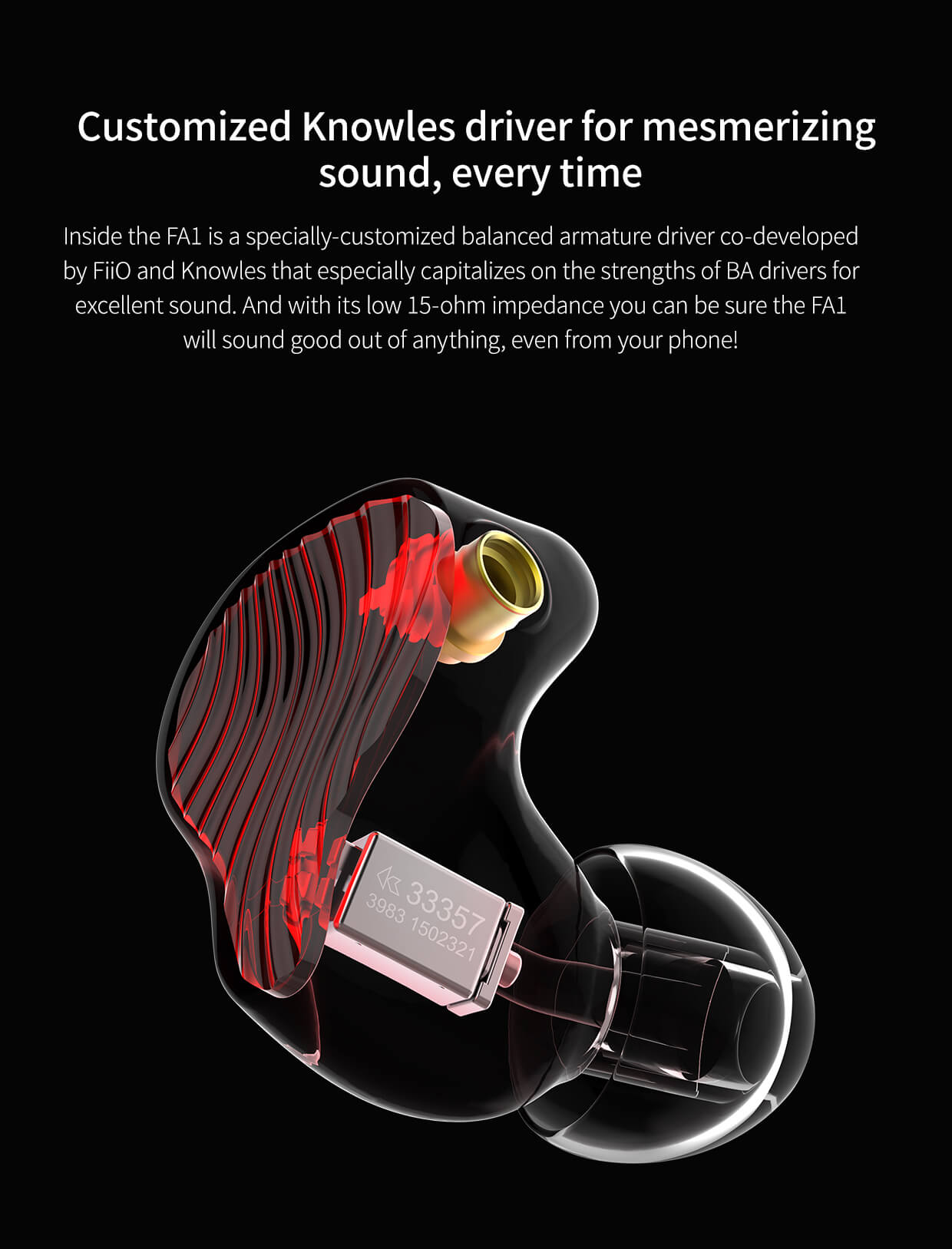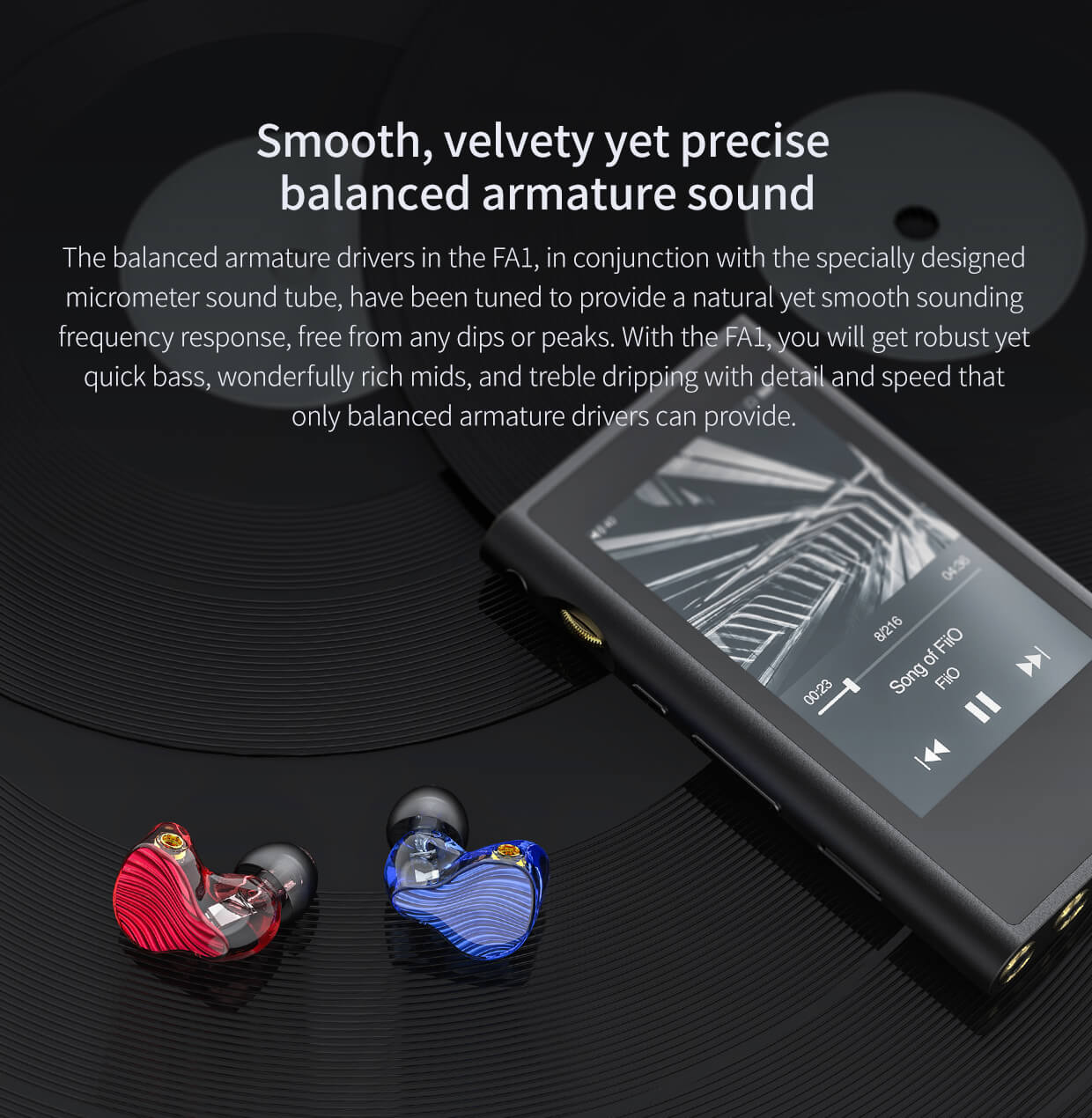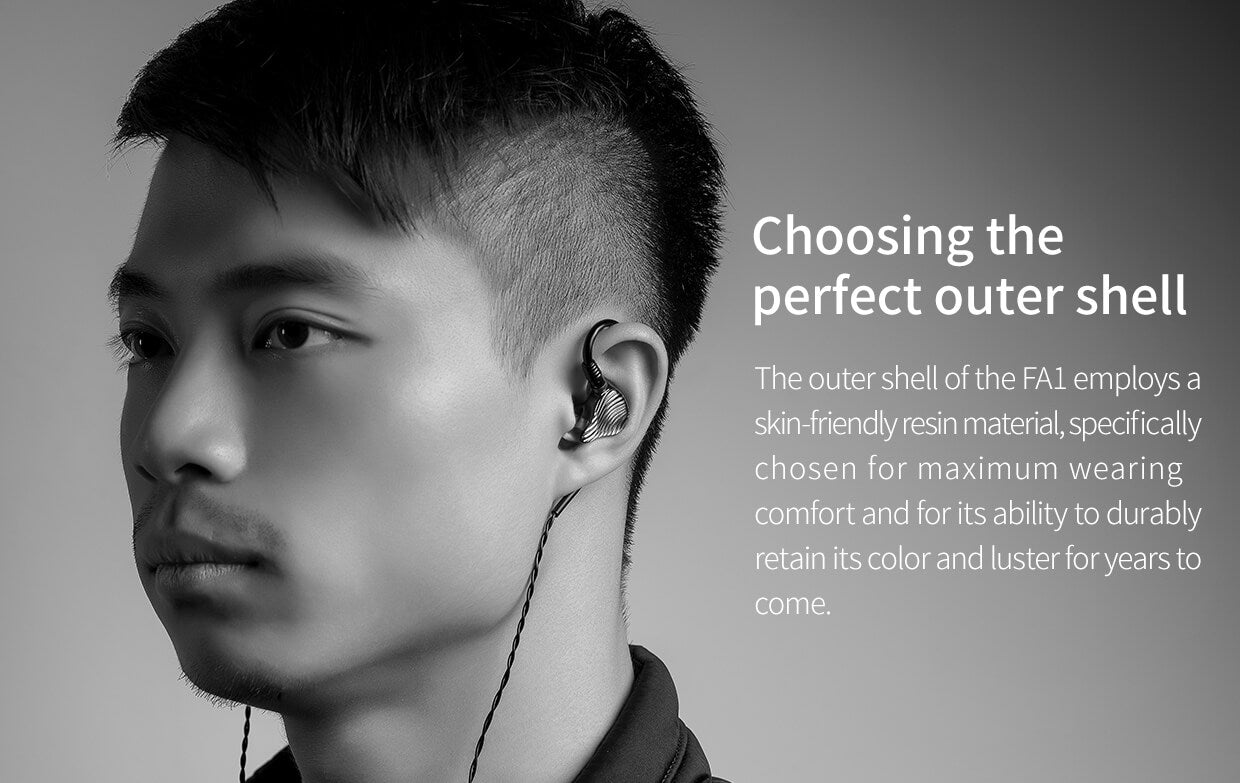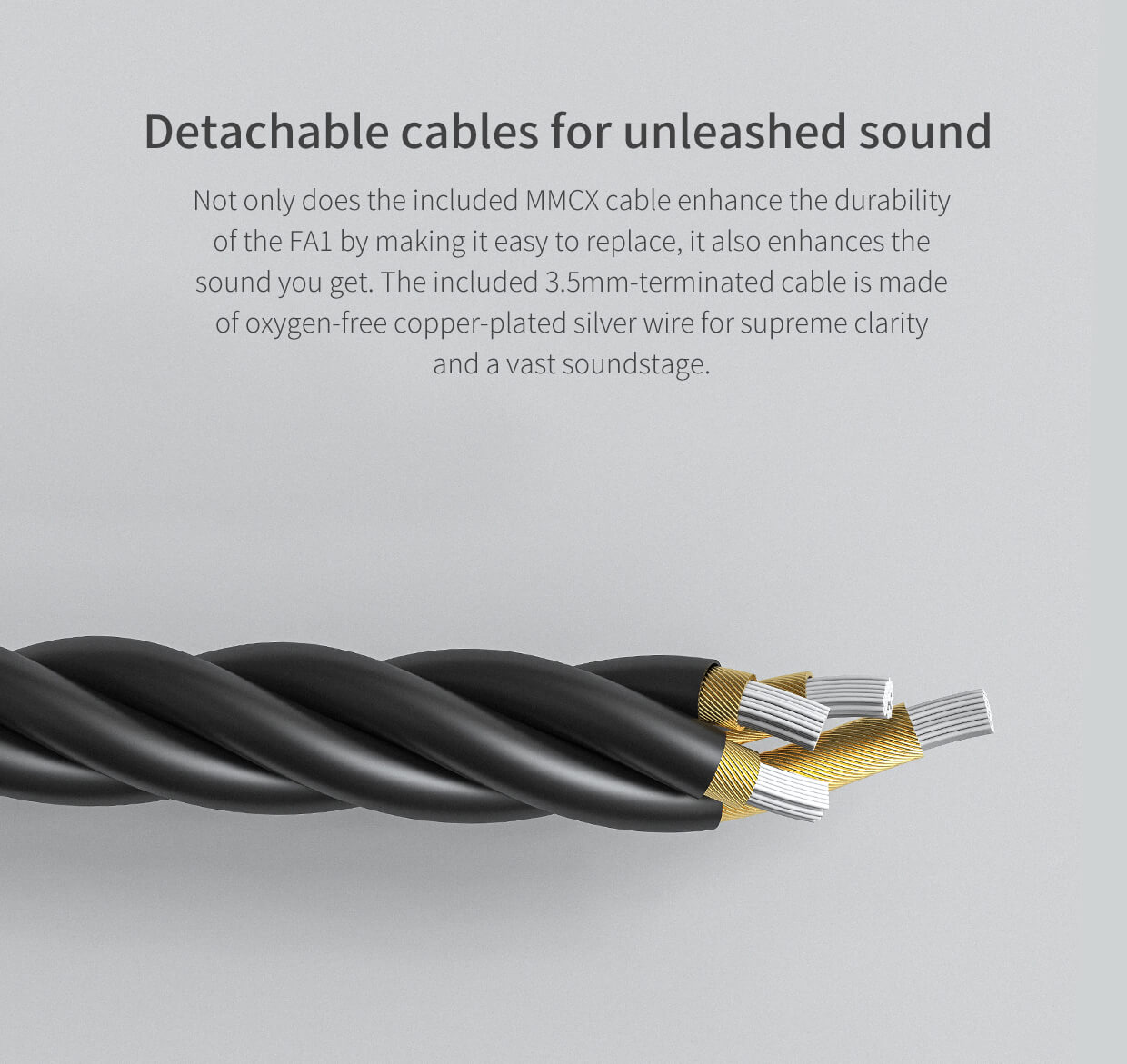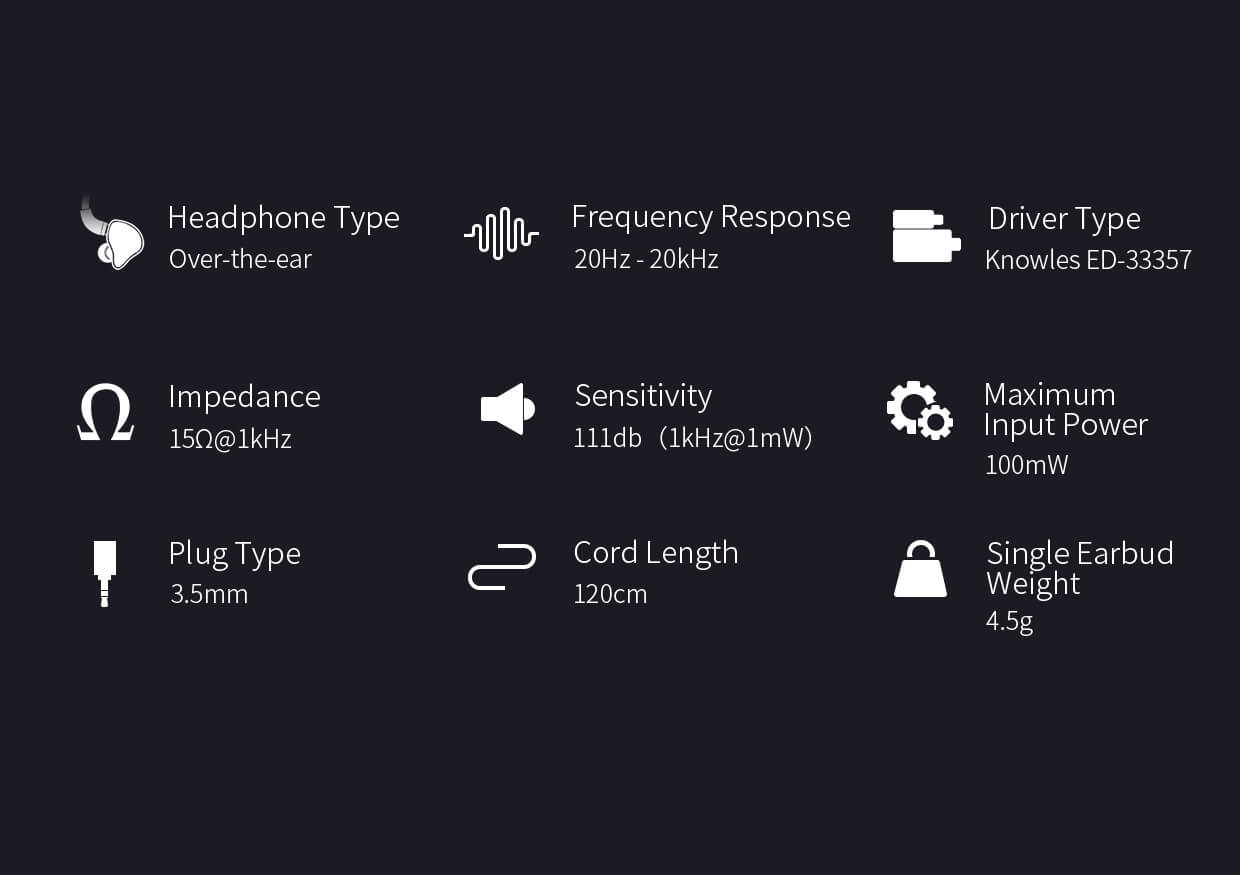## Customer Reviews

Based on 6 reviews